﻿ 多智能体系统的二部一致性问题研究

# 多智能体系统的二部一致性问题研究Research on Bipartite Consensus Problems of Multi-Agent Systems

Abstract: For a first-order discrete-time multi-agent system in which cooperation and competition mechanism coexist, we establish its bipartite consensus theories under undirected and directed topology graph based on graph theory, nonnegative matrix theory and stability theory through gauge transformation.

1. 引言

2. 预备知识

3. 无向拓扑图下的一阶离散系统的二部一致性

${x}_{i}\left(k+1\right)={x}_{i}\left(k\right)+{u}_{i}\left(k\right)$ (1)

${u}_{i}\left(k\right)=-\epsilon \underset{j\in {N}_{i}}{\sum }|{a}_{ij}|\left({x}_{i}\left(k\right)-\mathrm{sgn}\left({a}_{ij}\right){x}_{j}\left(k\right)\right)$ (2)

$x\left(k+1\right)={\left[\begin{array}{ccc}{x}_{1}\left(k+1\right)& {x}_{2}\left(k+1\right)& \begin{array}{cc}\cdots & {x}_{n}\left(k+1\right)\end{array}\end{array}\right]}^{\text{T}}$，则

$x\left(k+1\right)=x\left(k\right)-\epsilon Lx\left(k\right)=\left(I-\epsilon L\right)x\left(k\right)=\stackrel{^}{P}x\left(k\right)$ (3)

$z\left(k\right)=Dx\left(k\right),D\in {D}^{*}$ (4)

$\begin{array}{c}z\left(k+1\right)=Dx\left(k+1\right)=D\stackrel{^}{P}x\left(k\right)=D\stackrel{^}{P}{D}^{-1}z\left(k\right)=D\left(I-\epsilon L\right){D}^{-1}z\left(k\right)\\ =\left(I-\epsilon DL{D}^{-1}\right)z\left(k\right)=\left(I-\epsilon {L}_{D}\right)z\left(k\right)={P}_{d}z\left(k\right)\end{array}$ (5)

${l}_{D,ih}=\left\{\begin{array}{l}\underset{j\in {N}_{i}}{\sum }|{a}_{ij}|,h=i\\ {\sigma }_{i}{\sigma }_{h}{a}_{ih},h\ne i\end{array}$ (6)

(1) $G\left(A\right)$ 的所有环是正环；

(2) 存在 $D\in {D}^{*}$，使得 $DAD$ 是非负矩阵，且在规范变换下得到的新的Laplacian矩阵

${L}_{D}=\left[\begin{array}{cccc}\underset{j\in {N}_{1}}{\sum }|{a}_{1j}|& -|{a}_{12}|& \cdots & -|{a}_{1n}|\\ -|{a}_{21}|& \underset{j\in {N}_{2}}{\sum }|{a}_{2j}|& \cdots & -|{a}_{2n}|\\ ⋮& ⋮& \ddots & ⋮\\ -|{a}_{n1}|& -|{a}_{n2}|& \cdots & \underset{j\in {N}_{n}}{\sum }|{a}_{nj}|\end{array}\right]$ 是行和为零的对角占优矩阵。

(3) 0是L的特征值。

(1) $G\left(A\right)$ 有一个或多个环是负的；

(2) 不存在 $D\in {D}^{*}$，使得 $DAD$ 是非负的；

(3) L的所有特征值大于零，即 $\varphi \left(x\right)>0$

${G}_{i}=\left\{s||s-{a}_{ii}|\le {R}_{i},s\in \overline{)C}\right\}$$i=1,2,\cdots ,n$ 为矩阵A的第i个Gershgorin圆，并称 ${R}_{i}$ 为Gershgorin圆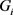的半径。

Perron矩阵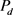的参数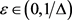，则矩阵具有如下性质：

(1)是一个非负行随机矩阵，并且有一个平凡特征值1；

(2)的所有特征值均在一个单位圆内；

(3) 若是平衡的，则是一个双随机矩阵；

(4) 若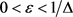，则是一个本原矩阵。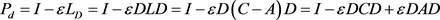(7)

(2) 令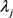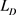的第j个特征值，则的第j个特征值为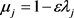。由Gershgorin定理，的所有特征值在圆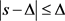内，令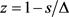，则有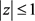，即的所有特征值均在一个单位圆内。

(3) 若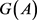是一个平衡图，则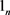的左特征向量，即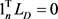，可得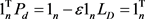，这说明的列和为1。又由(1)知，是一个非负行随机矩阵，所以是一个双随机矩阵。

(4) 若是强连通的，则是一个不可约矩阵。对任意，令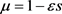，将圆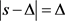映射到严格位于单位圆内的圆，其中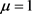，这说明在时只有一个模长为1的单特征值，可得是一个本原矩阵。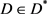是规范变换中使得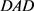非负的矩阵，则系统(1)的二部一致的解为。若不是结构平衡的，则对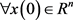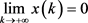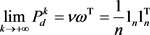(8)(9)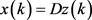，得：(10)不是结构平衡的，则为严格对角占优矩阵。由引理1 (2)知，此时的所有特征值均在一

4. 有向拓扑图下一阶离散系统的二部一致性

(1)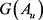是结构平衡的；

(2)的所有环是正环；

(3) 存在，使得是非负矩阵；

(4) 0是L的特征值。

(1)不是结构平衡的；

(2)至少有一个负有向环；

(3) 不存在，使得是非负矩阵。是结构平衡的，令v是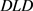的一个非零左特征向量，且满足，结合定理1建立有向拓扑下的二部一致性理论。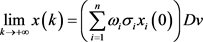。若是加权平衡的，则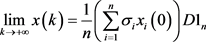。若不是结构平衡的，则对.，显然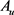对应的符号图是一个无向连通图，则由定理1类似可得，系统(1)可获得二部一致性。引理4 (3)保证了D的存在，由定理1知，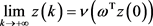，由，可得：(11)是加权平衡的，则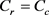，因此A为对称矩阵，此时A对应的符号图是无向图，系统(1)在协议(2)下取得的二部一致的解即为定理1中的结果.若不是结构平衡的，由引理1 (2)知，此的所有特征值均在一个单位圆内，因此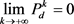。由，有：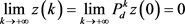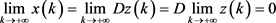.

5. 有向拓扑图下一阶离散系统的二部一致性

 DeGroot, M.H. (1974) Reaching a Consensus. Journal of the American Statistical Association, 69, 118-121.
https://doi.org/10.1080/01621459.1974.10480137

 Li, B., Li, J. and Huang, K.W. (2013) Modeling and Flocking Consensus Analysis for Large-Scale UAV Swarms. Mathematical Problems in Engineering, 2013, Article ID: 368369.
https://doi.org/10.1155/2013/368369

 Shi, H., Wang, L. and Chu, T. (2009) Flocking of Multi-Agent Systems with a Dynamic Virtual Leader. International Journal of Control, 82, 43-58.
https://doi.org/10.1080/00207170801983091

 Benediktsson, J.A. and Swain, P.H. (1992) Consensus Theoretic Classification Methods. IEEE Transactions on Systems Man & Cybernetics, 22, 688-704.
https://doi.org/10.1109/21.156582

 Reynolds, C.W. (1987) Flocks, Herds and Schools: A Distributed Behavioral Model. ACM Siggrapy Computer Graphics, 21, 25-34.
https://doi.org/10.1145/37402.37406

 Vicsek, T., Czir, D.K.A., Ben-Jacob, E., et al. (1995) Novel Type of Phase Transition in a System of Self-Driven Particles. Physical Review Letters, 75, 1226-1229.
https://doi.org/10.1103/PhysRevLett.75.1226

 Jiang, Y., Zhang, H. and Chen, J. (2016) Sign-Consensus of Linear Multi-Agent Systems over Signed Directed Graphs. IEEE Transactions on Industrial Electronics, 64, 5075-5083.
https://doi.org/10.1109/TIE.2016.2642878

 Hu, J. and Zhu, H. (2015) Adaptive Bipartite Consensus on Coopetition Networks. Physica D: Nonlinear Phenomena, 307, 14-21.
https://doi.org/10.1016/j.physd.2015.05.012

 Altafini, C. (2012) Consensus Problems on Networks with Antagonistic Interactions. IEEE Transactions on Automatic Control, 58, 935-946.
https://doi.org/10.1109/TAC.2012.2224251

 Zhou, Y. and Hu, J. (2013) Event-Based Bipartite Consensus on Signed Networks. IEEE International Conference on Cyber Technology in Automation, Control and Intelligent Systems, Nanjing, 26-29 May 2013, 326-330.
https://doi.org/10.1109/CYBER.2013.6705467

 Yang, D., Ren, W., Liu, X., et al. (2016) Decentralized Event-Triggered Consensus for Linear Multi-Agent Systems under General Directed Graphs. Automatica, 69, 242-249.
https://doi.org/10.1016/j.automatica.2016.03.003

 Zhang, H. and Chen, J. (2017) Bipartite Consensus of Multi-Agent Systems over Signed Graphs: State Feedback and Output Feedback Control Approaches. International Journal of Robust and Nonlinear Control, 27, 3-14.
https://doi.org/10.1002/rnc.3552

 Olfati-Saber, R., Fax, J.A. and Murray, R.M. (2007) Consensus and Cooperation in Networked Multi-Agent Systems. Proceedings of the IEEE, 95, 215-233.
https://doi.org/10.1109/JPROC.2006.887293

 Franklin, J.N. (2012) Matrix Theory. Courier Corporation, North Chelmsford.

Top